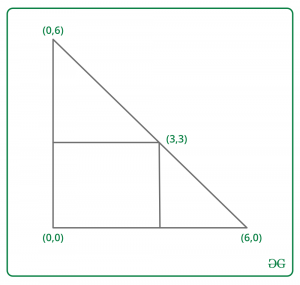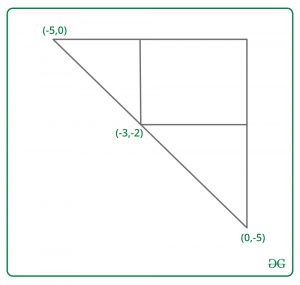# Find two vertices of an isosceles triangle in which there is rectangle with opposite corners (0, 0) and (X, Y)

• Last Updated : 22 Mar, 2021

Given two integers X and Y. The task is to find two vertices of an isosceles triangle ABC(right-angled at B) which has one vertex at a point B(0, 0). And there is a rectangle with opposite sides (0, 0) and (X, Y). All the points of this rectangle are located inside or on the border of the triangle. Print 4 integers x1, y1, x2, y2, where A(x1, y1) and B(x2, y2).
Examples:

Input : X = 3, Y = 3
Output : 6 0 0 6Input : X = -3, y = -2
Output : -5 0 0 -5Approach :
Let Val = |x| + |y|. Then the first point is (Val * sign(x), 0) and the second point is (0, Val * sign(y)).
Let’s see how it works for x > 0 and y > 0. Other cases can be proved in a similar way.
We need to show, that (x, y) belongs to our triangle(including its borders). In fact (x, y) belongs to segment, connecting (x + y, 0) with (0, x + y). The line through (x + y, 0) and (0, x + y) is Y = – X + x + y. Using coordinates (x, y) in this equation proves our answer.
Below is the implementation of the above approach:

## C++

 `// C++ program to find two vertices of an``// isosceles triangle in which there is``// rectangle with opposite side (0, 0) and (x, y)``#include ``using` `namespace` `std;` `// Function to find two vertices of an``// isosceles triangle in which there is``// rectangle with opposite side (0, 0) and (x, y)``int` `Vertices(``int` `x, ``int` `y)``{``    ``// Required value;``    ``int` `val = ``abs``(x) + ``abs``(y);` `    ``// print x1 and y1``    ``cout << val * (x < 0 ? -1 : 1) << ``" 0 "``;` `    ``// print x2 and y3``    ``cout << ``"0 "` `<< val * (y < 0 ? -1 : 1);``}` `// Driver code``int` `main()``{``    ``int` `x = 3, y = 3;` `    ``// Function call``    ``Vertices(x, y);` `    ``return` `0;``}`

## Java

 `// Java program to find two vertices of an``// isosceles triangle in which there is``// rectangle with opposite side (0, 0) and (x, y)``class` `GFG``{` `// Function to find two vertices of an``// isosceles triangle in which there is``// rectangle with opposite side (0, 0) and (x, y)``static` `void` `Vertices(``int` `x, ``int` `y)``{``    ``// Required value;``    ``int` `val = Math.abs(x) + Math.abs(y);` `    ``// print x1 and y1``    ``System.out.print(val * (x < ``0` `? -``1` `: ``1``) + ``" 0 "``);` `    ``// print x2 and y3``    ``System.out.print(``"0 "` `+ val * (y < ``0` `? -``1` `: ``1``));``}` `// Driver code``public` `static` `void` `main(String[] args)``{``    ``int` `x = ``3``, y = ``3``;` `    ``// Function call``    ``Vertices(x, y);``}``}` `// This code is contributed by Rajput-Ji`

## Python3

 `# Python3 program to find two vertices of an``# isosceles triangle in which there is``# rectangle with opposite side (0, 0) and (x, y)` `# Function to find two vertices of an``# isosceles triangle in which there is``# rectangle with opposite side (0, 0) and (x, y)``def` `Vertices(x, y) :` `    ``# Required value;``    ``val ``=` `abs``(x) ``+` `abs``(y);` `    ``# print x1 and y1``    ``if` `x < ``0` `:``        ``x ``=` `-``1``    ``else` `:``        ``x ``=` `1``        ` `    ``print``(val ``*` `x,``"0"``,end ``=` `" "``);` `    ``# print x2 and y3``    ``if` `y < ``0` `:``        ``y ``=` `-``1``    ``else` `:``        ``y ``=` `1``    ` `    ``print``(``"0"``,val ``*` `y);`  `# Driver code``if` `__name__ ``=``=` `"__main__"` `:` `    ``x ``=` `3``; y ``=` `3``;` `    ``# Function call``    ``Vertices(x, y);` `# This code is contributed by AnkitRai01`

## C#

 `// C# program to find two vertices of an``// isosceles triangle in which there is``// rectangle with opposite side (0, 0) and (x, y)``using` `System;``    ` `class` `GFG``{` `// Function to find two vertices of an``// isosceles triangle in which there is``// rectangle with opposite side (0, 0) and (x, y)``static` `void` `Vertices(``int` `x, ``int` `y)``{``    ``// Required value;``    ``int` `val = Math.Abs(x) + Math.Abs(y);` `    ``// print x1 and y1``    ``Console.Write(val * (x < 0 ? -1 : 1) + ``" 0 "``);` `    ``// print x2 and y3``    ``Console.Write(``"0 "` `+ val * (y < 0 ? -1 : 1));``}` `// Driver code``public` `static` `void` `Main(String[] args)``{``    ``int` `x = 3, y = 3;` `    ``// Function call``    ``Vertices(x, y);``}``}` `// This code is contributed by Rajput-Ji`

## Javascript

 ``
Output:
`6 0 0 6`

Time Complexity : O(1)

My Personal Notes arrow_drop_up